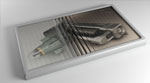It could be compared to the VBA language inside EXCEL and the logic is indeed the same but the code is of course different. After creating the MAXScript and running it the panels of the advertisement would be animated in a certain step where they are smoothly rotating on one side and then on another and so changing the actual image which is placed on the faces of the panels. At the end we will use the 3D-Sphere material library which you can download from the menu on the right side of 3D-Sphere and apply the materials and textures to our model. For rendering I will use Photometric Lights and MentalRay. You can download the reference materials, the used MAXScript and as well watch the tutorial below and if you have any questions, just write us.

• Creating an animation in 3ds Max
• Using poly-modelling technique
• Using MAXScript to do the animation
• Using Photometric lights
• Rendering using MentalRay

• 01:00:00

• 3ds Max 2012

```for i=1 to 27 do
(
if i<10 then panel="Panel00" else panel="Panel0"
a= i as string
theObject=Execute("\$'"+panel+a)
select theObject
slide = num as string + "f"
slide2 = slide as time
sliderTime=slide2
rotate \$ (angleaxis 0 [0,0,1])
slide3= num2 as string + "f"
slide4 = slide3 as time
sliderTime=slide4
rotate \$ (angleaxis 120 [0,0,1])
num=num+2
num2=num2+2
clearSelection()
)
)
```